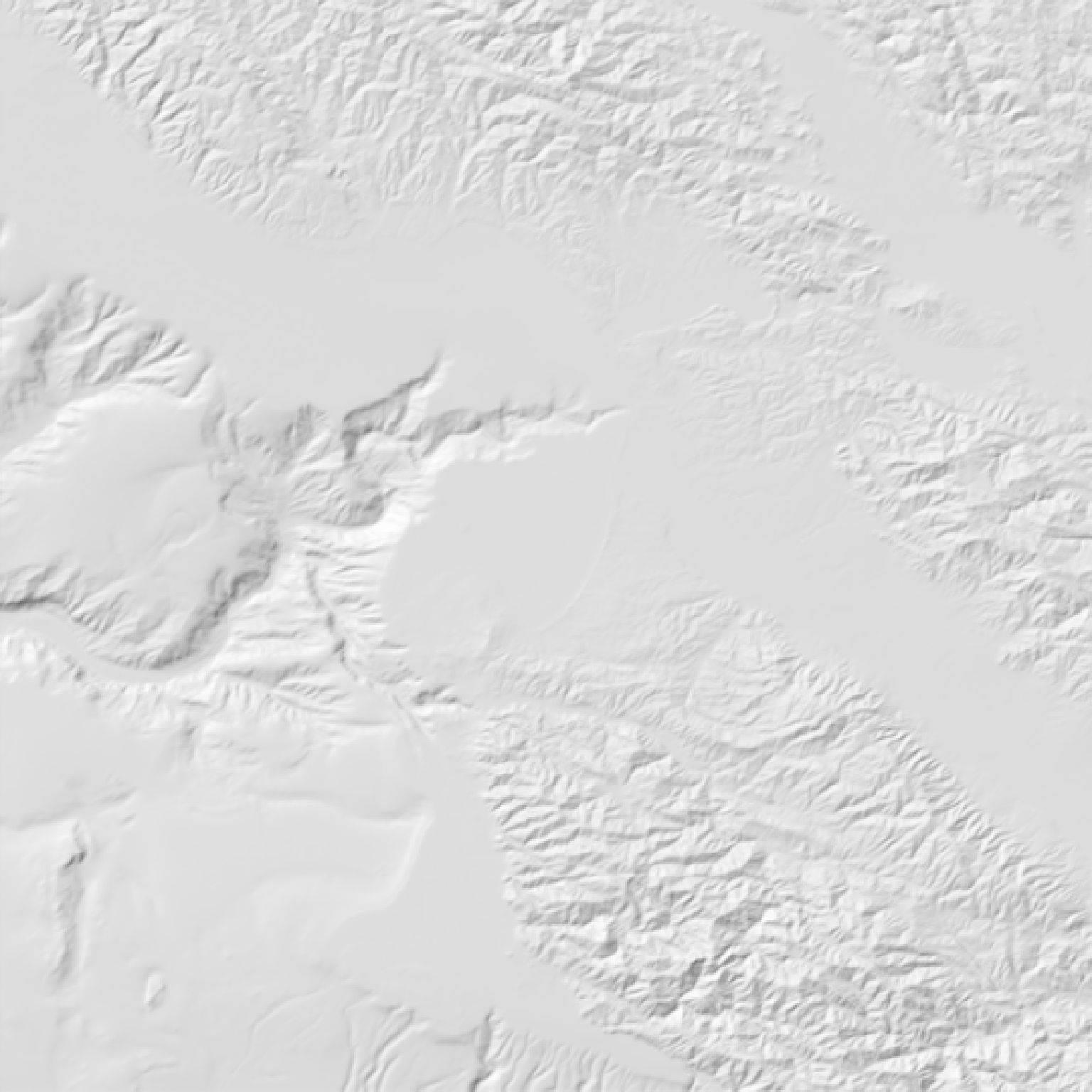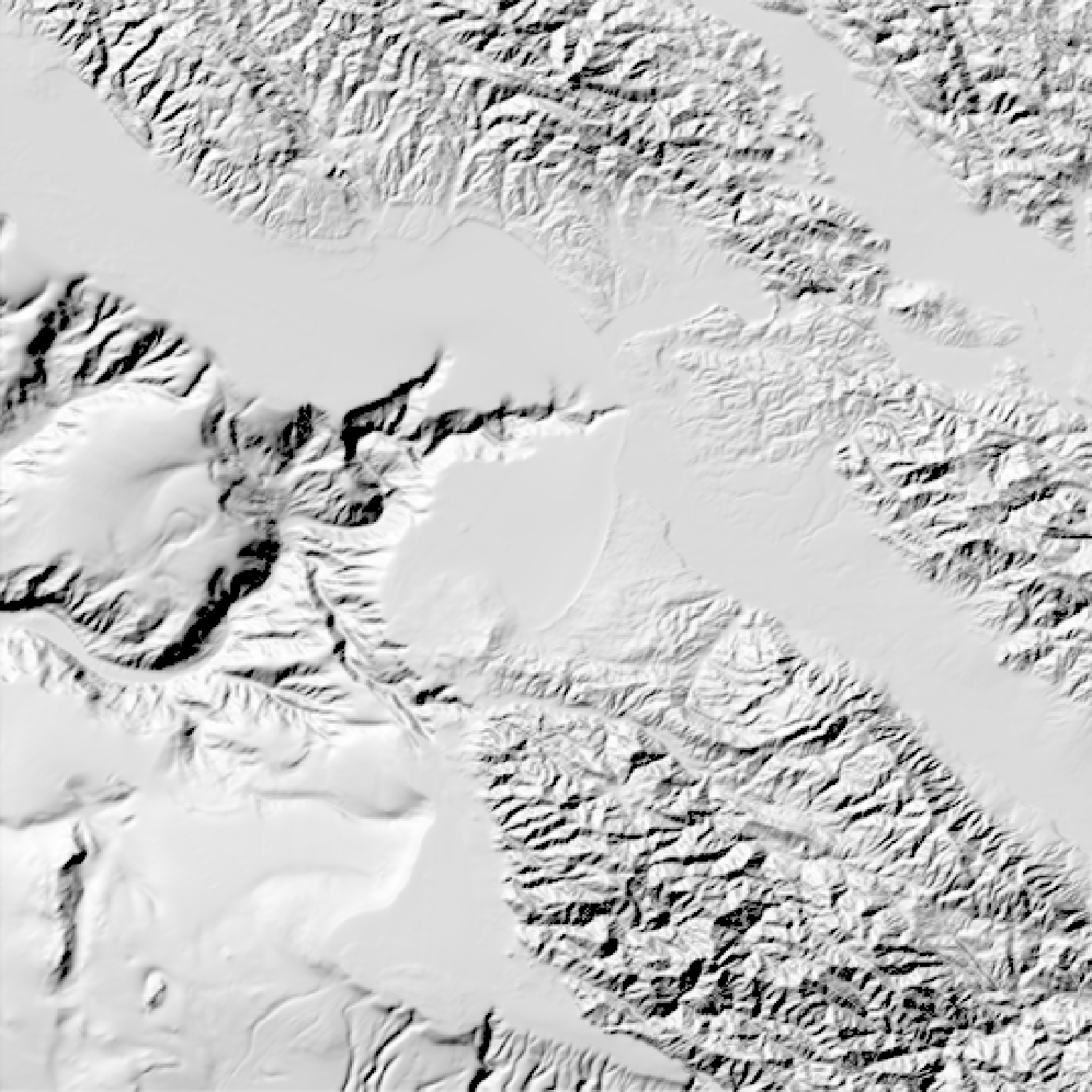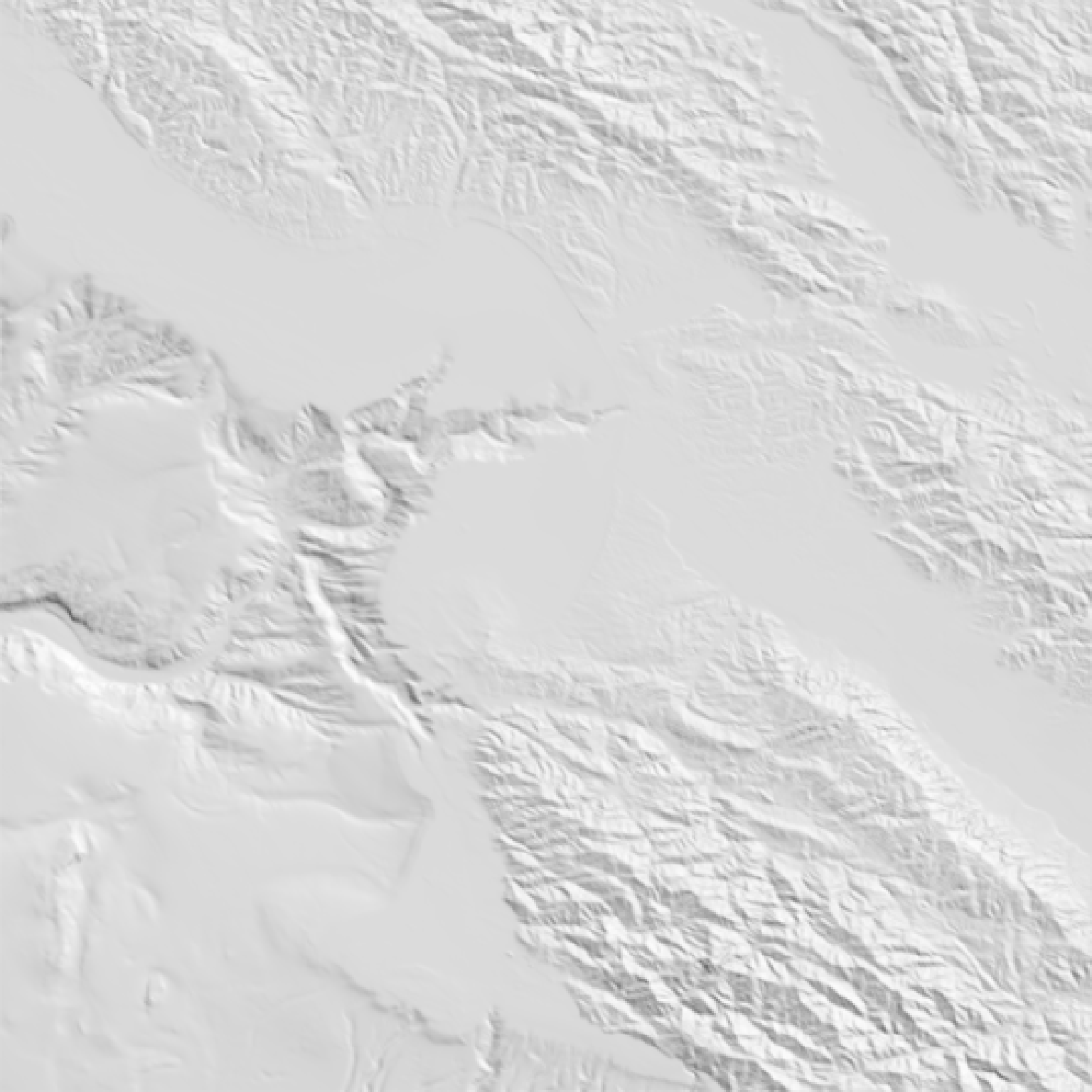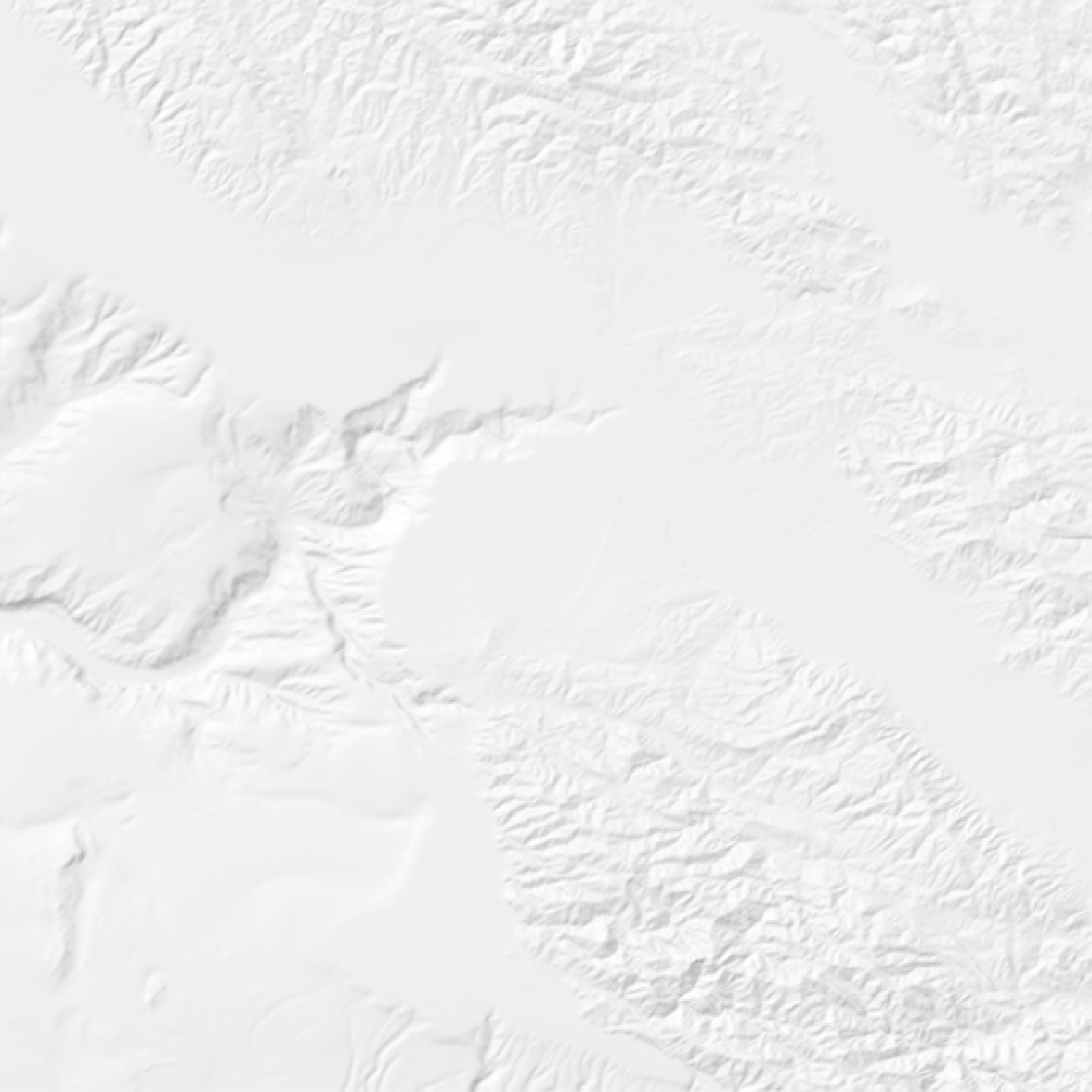Calculates local shadow map for a elevation matrix by calculating the dot product between light direction and the surface normal vector at that point. Each point's intensity is proportional to the cosine of the normal vector.

lamb_shade(
heightmap,
sunaltitude = 45,
sunangle = 315,
zscale = 1,
zero_negative = TRUE
)

## Arguments

heightmap

A two-dimensional matrix, where each entry in the matrix is the elevation at that point. All points are assumed to be evenly spaced.

sunaltitude

Default 45. The azimuth angle as measured from the horizon from which the light originates.

sunangle

Default 315 (NW). The angle around the matrix from which the light originates.

zscale

Default 1. The ratio between the x and y spacing (which are assumed to be equal) and the z axis.

zero_negative

Default TRUE. Zeros out all values below 0 (corresponding to surfaces facing away from the light source).

## Value

Matrix of light intensities at each point.

## Examples

if(rayshader:::run_documentation()) {
montereybay %>%
plot_map()
}#Increase the intensity by decreasing the zscale
montereybay %>%
plot_map()
}#Change the sun direction
montereybay %>%